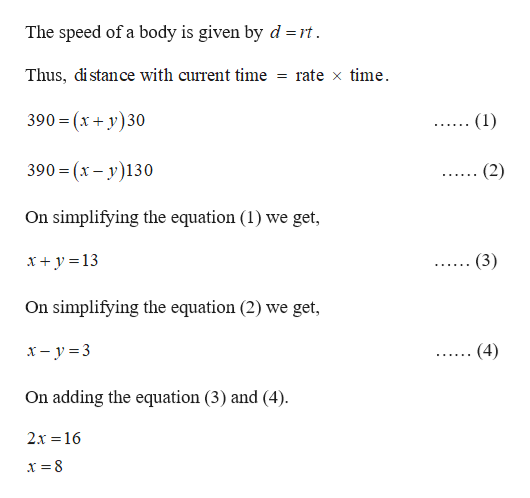# Two runners begin at the same point on a 390-m circular track and run at different speeds. If they run in opposite directions, they pass each other in 30 sec. If they run in the same direction, they meet each other in 130 sec. Find the speed of each runner.

Question
2 views

Two runners begin at the same point on a 390-m circular track and run at different speeds. If they run in opposite directions, they pass each other in 30 sec. If they run in the same direction, they meet each other in 130 sec. Find the speed of each runner.

check_circle

Step 1

Let x represents the speed of the one runner and y rep...help_outlineImage TranscriptioncloseThe speed of a body is given by d =rt. = rate x time. Thus, distance with current time 390 = (x + y)30 (1) 390 = (x – y)130 (2) .... On simplifying the equation (1) we get, (3) x+ y =13 On simplifying the equation (2) we get, x- y = 3 (4) ...... On adding the equation (3) and (4). 2x =16 x = 8 fullscreen

### Want to see the full answer?

See Solution

#### Want to see this answer and more?

Solutions are written by subject experts who are available 24/7. Questions are typically answered within 1 hour.*

See Solution
*Response times may vary by subject and question.
Tagged in
MathAlgebra

### Other